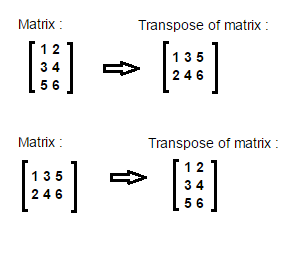### Matrix Transpose program in java

Hi! we will learn how to transpose a matrix in java.Main logic behind matrix transpose is:
//transpose matrix
int transpose[][] = new int[columns][rows];
for (int i = 0; i < rows; i++) {
for (int j = 0; j < columns; j++)
transpose[j][i] = matrix[i][j];
}

Example/ Full Program/SourceCode to do Matrix Transpose in java >
 package matrix; import java.util.Scanner; /** Copyright (c), AnkitMittal  JavaMadeSoEasy.com - Matrix Transpose in java */ public class TransposeMatrix {    public static void main(String...args) {           Scanner scanner = new Scanner(System.in);           System.out.println("Enter number of rows in matrix : ");           int rows = scanner.nextInt();           System.out.print("Enter number of columns in matrix : ");           int columns = scanner.nextInt();           int matrix[][] = new int[rows][columns];           System.out.println("Enter the elements in matrix :");           for (int i = 0; i < rows; i++) {                  for (int j = 0; j < columns; j++) {                        matrix[i][j] = scanner.nextInt();                  }           }                     //transpose matrix           int transpose[][] = new int[columns][rows];           for (int i = 0; i < rows; i++) {                  for (int j = 0; j < columns; j++)                        transpose[j][i] = matrix[i][j];           }           System.out.println("\nEntered Matrix is : ");           for (int i = 0; i < rows; i++) {                  for (int j = 0; j < columns; j++) {                        System.out.print(matrix[i][j] + " ");                  }                  System.out.println();           }                     System.out.println("\nTranspose of entered matrix is : ");           for (int i = 0; i < columns; i++) {                  for (int j = 0; j < rows; j++)                        System.out.print(transpose[i][j] + " ");                  System.out.println();           }    } } /*OUTPUT Enter number of rows in matrix : 3 Enter number of columns in matrix : 2 Enter the elements in matrix : 1 2 3 4 5 6 Entered Matrix is : 1 2 3 4 5 6 Transpose of entered matrix is : 1 3 5 2 4 6 */

So we learned program to do Matrix Transpose in java.

Having any doubt? or you you liked the tutorial! Please comment in below section.